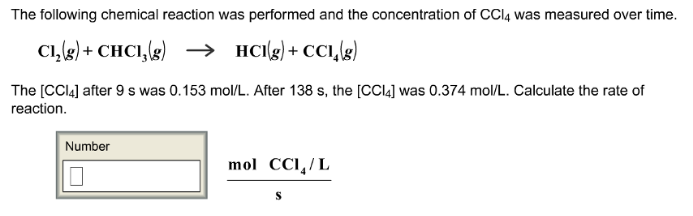# Problem: The following chemical reaction was performed and the concentration of CCl4 was measured over time. Cl2 (g) + CHCl3 (g) → HCl (g) + CCI (g) The [CCl4] after 9 s was 0.153 mo/L. After 138 s, the [CCl4] was 0.374 mol/L. Calculate the rate of reaction.

###### FREE Expert Solution
97% (448 ratings)###### Problem Details

The following chemical reaction was performed and the concentration of CCl4 was measured over time.

Cl2 (g) + CHCl3 (g) → HCl (g) + CCI (g)

The [CCl4] after 9 s was 0.153 mo/L. After 138 s, the [CCl4] was 0.374 mol/L. Calculate the rate of reaction.